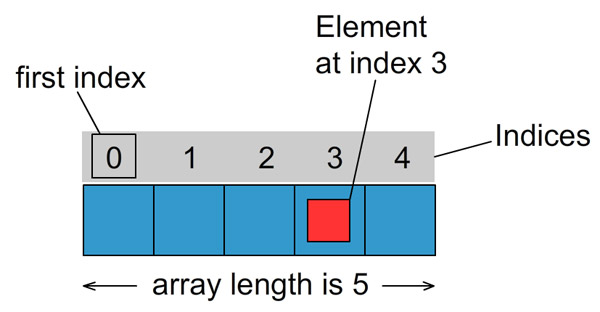Java Array

In this tutorial I will show you how to create and use arrays in Java

The array is a collection of variables from the same type. Arrays are used for multiple purposes. For example you may want to store all prices in a shop in one array. But what makes arrays really useful is the way you can work with the values stored into it.

The genaral form to declare a new array in java is as follows:

type arrayName[] = new type[numberOfElements];

where type is a primitive type (read more about primitive types in this tutorial) or Object. numberOfElements is the number of elements you will store into the array. This value can’t change. Java does not support dynamic arrays. If you need a flexible and dynamic structure for holding objects you may want to use some of the Java collections. For more details reed Java Collections Tutorial.

Overview

Java supports one-dimensional or multy-dimensional arrays.Each item in an array is called an element, and each element is accessed by its numerical index. As shown in the preceding illustration, numbering begins with 0. The 4th element, for example, would therefore be accessed at index 3.

Initialize Array

Lets create an array to store the salaries of all employees in a small company of 5 people.

int salaries[] = new int;

The type of the array (in this case int) applies to all values in the array. You can not mix types in one array.

Put Values into Array

Now that we have our salaries array initialized we want to put some values into it. We can do this either during the initialization like this:

int salaries[] = {50000, 75340, 110500, 98270, 39400};

or do it at a later point like this:

int salaries[] = new int;
salaries = 50000;
salaries = 75340;
salaries = 110500;
salaries = 98270;
salaries = 39400;

Iterate over Arrays

You can work with the value of specific element by calling it like this:

System.out.println("The value of the 4th element in the array is " + salaries);

This will produce the output:

The value of the 4th element in the array is 98270

or you can iterate over the values of all elements in the array using for loop or while loop. Reed more about loops in our previous tutorial Java Loops

public class ArrayExample {
public static void main(String[] args) {
int salaries[] = {50000, 75340, 110500, 98270, 39400};
for(int i=0; i<salaries.length; i++) {
System.out.println("The element at index " + i + " has the value of " + salaries[i]);
}
}
}

The output the program above produces is:

The element at index 0 has the value of 50000
The element at index 1 has the value of 75340
The element at index 2 has the value of 110500
The element at index 3 has the value of 98270
The element at index 4 has the value of 39400

Note the use of salaries.length . Arrays in java have the length property which returns the length of the array.